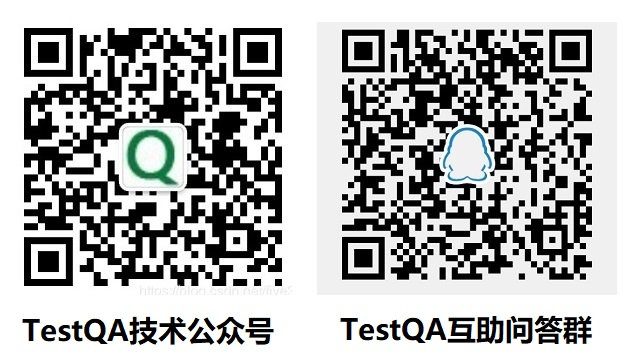# 论接口自动化测试方法

• 0 推荐
• 0 收藏，859 浏览

## 动态库接口测试

``````import unittest
from ctypes import *

class TestMathDLL(unittest.TestCase):
def __init__(self):
super().__init__()
self.dll = CDLL("math.dll")

def test_add(self):
actual = self.dll.add(2, 9)
expect = 11
self.assertEqual(actual, expect)

if __name__ == '__main__':
unittest.main()
``````

## 服务接口测试

``````import unittest
import requests

class TestMathDLL(unittest.TestCase):
def __init__(self):
super().__init__()
self.base_url = 'http://localhost/math'

def test_add(self):
add_url = '/add'
data = {'num1': 2, 'num2': 8}

actual = requests.post(self.base_url + add_url, data=data).json()
expect = {'success': True, 'result': 10}

self.assertEqual(actual, expect)

if __name__ == '__main__':
unittest.main()``````

## 接口对比测试

• 本质上是无用例测试
• 特别适合重构验证和回归测试
• 可作为预上线环境的测试验收方式TestQA's Blog